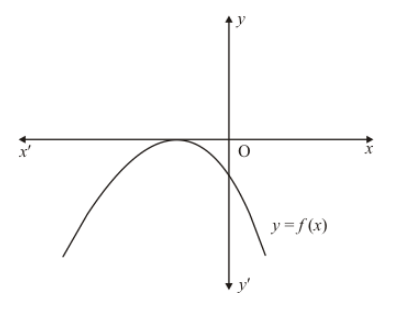# In Q. No. 15, write the sign of c.`
Question:

In Q. No. 15, write the sign of c.

Solution:

The parabola $y=a x^{2}+b x+c$ cuts $y$-axis at P which lies on OY.Putting $x=0$ in $y=a x^{2}+b x+c$, we get $y=c$. So the coordinates of $\mathrm{P}$ are $(0, c)$. Clearly, $\mathrm{P}$ lies on $O Y^{\prime}$. Therefore $c<0$